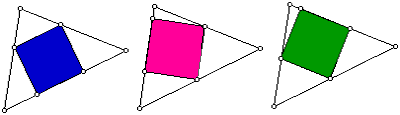# Inscribed Square

Consider the four vertices of a square. To have a square inscribed in a triangle, two of the square’s vertices must lie on the same side of the triangle. Here are some examples:Given a triangle, can we construct an inscribed square? All inscribed squares?Extension
For the triangle shown above, there are three distinct inscribed squares. What conjectures can you make about number of inscribed squares for a given triangle? Is there any relationship between the area of the inscribed square and the given triangle?